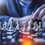So. Let's say 1 =.999 repeating (my math proof)
Let's also say x =.999 repeating We multiply each side by 10 to get
10x = 9.999 repeating
Subtract x from each side and since we said x = .999 repeating...
9x = 9
x = 1 = .999
You might have already been aware of this concept.
Here is another one,
1/3 =.333...
2/3 =.666...
3/3 = .999...
1 = .999...

Topic 2,
2 = 1
Now, this one has an error in it, let's see if you can find it.
2 = 1
a = b
a^2 = ab (a = b as said on the second statement)
a^2 - b^2 = ab - b^2
Now I am going to factorize
(a+b)(a-b) = b(a-b)
Simplify
a + b = b
Since a = b
b + b = b
Subsitute b for 1,
1 + 1 = 1
2 = 1

Now, in math class, you might have heard about you can't divide by 0.
Now, why is that?
Let's say one person says Ummm.... x/0 = 0.
So now let's prove that wrong.
What do you get if
12 no?
12/.1 = 120
12/.01 = 1200
12/.001 = 12000
12/.0001 = 120000
and so on.
What will happen if we reach 0? It will keep on getting smaller and smaller and smaller for infinite possibilities. We subtract infinity and we finally reach 0. What is 12/0? That's like saying what's 0 * x = 12. No matter what you do it will never be 12. Then another guy says, hey! When x/0, x = 1! He feels very smart of himself but then you just say, does 1*0 = 12? This is why dividing by 0 is undefined. Thanks for reading, I will be sure to add more notes later on. Thanks for stopping by, be sure to point out the mistake in example # 2.Note by Mathmetician Scientist
1 year, 10 months ago

This discussion board is a place to discuss our Daily Challenges and the math and science related to those challenges. Explanations are more than just a solution — they should explain the steps and thinking strategies that you used to obtain the solution. Comments should further the discussion of math and science.

When posting on Brilliant:

• Use the emojis to react to an explanation, whether you're congratulating a job well done , or just really confused .
• Ask specific questions about the challenge or the steps in somebody's explanation. Well-posed questions can add a lot to the discussion, but posting "I don't understand!" doesn't help anyone.
• Try to contribute something new to the discussion, whether it is an extension, generalization or other idea related to the challenge.

MarkdownAppears as
*italics* or _italics_ italics
**bold** or __bold__ bold
- bulleted- list
• bulleted
• list
1. numbered2. list
1. numbered
2. list
Note: you must add a full line of space before and after lists for them to show up correctly
paragraph 1paragraph 2

paragraph 1

paragraph 2

[example link](https://brilliant.org)example link
> This is a quote
This is a quote
    # I indented these lines
# 4 spaces, and now they show
# up as a code block.

print "hello world"
# I indented these lines
# 4 spaces, and now they show
# up as a code block.

print "hello world"
MathAppears as
Remember to wrap math in $$ ... $$ or $ ... $ to ensure proper formatting.
2 \times 3 $2 \times 3$
2^{34} $2^{34}$
a_{i-1} $a_{i-1}$
\frac{2}{3} $\frac{2}{3}$
\sqrt{2} $\sqrt{2}$
\sum_{i=1}^3 $\sum_{i=1}^3$
\sin \theta $\sin \theta$
\boxed{123} $\boxed{123}$

Sort by:

For Topic 2, it's the third line that's wrong.

- 1 year, 2 months ago